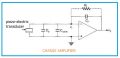# How to make parallel piezoelectric/PVDF transducers for force measurement?

#### safiana

Joined Jan 16, 2023
2
Hello everyone!I am having difficulty in understanding a concept since I am not in the electrical field. I am working on a project to measure the dynamic force on a surface using embedded PVDF transducers. Based on the theories I studied so far, if I connect this PVDF to the charge amplifier, the output voltage is only dependent on the feedback capacitor of the charge amplifier as follows:Where 1/cf is the charge amplifier gain that I put it on 0.1 mV/pC and d33 is piezoelectruc charge constant around 20 pC/N. I want to measure the dynamic force F through the measured voltage signal in the experiment. The problem is that I have two pieces of PVDF to measure the force and I have to connect them together (series or parallel I don't know) to measure the voltage and force from one channel. The experiment is like below where two pieces of PVDF are bonded between two metallic material.How can I connect these PVDF materials to measure the force through one channel? I tested the signal from each one and the voltage signal was -0.3 to 0.3 V. Both PVDF separately gave me same signal but with a phase shift because the load passes through the top surface in horizontal direction.

If I put these PVDF in parallel (all positives together / negatives together,) the voltage drops to 0.1 V. I didn't expect this and I thought I should see around 0.6 V. Then I put them in series and voltage became about 1-1.2 V but the appearance of the signal was not something I expected. I expect to see a sinusoidal voltage signal but that one was a noise signal with sudden jumps in its amplitude.

I would like to understand if I am doing something wrong here, or if generally this approach is possible, or I have to measure force from one PVDF and them multiply it by two.

Should I use the maxum amplitude to put in equation or peak to peak? I mean for each PVDF is my V value in equation 0.3 V or 0.6 V?

PVDF datasheet:
https://www.te.com/commerce/Documen...EnglishENG_DS_FDT_Series_A1.pdfCAT-PFS0008

#### ericgibbs

Joined Jan 29, 2010
17,180
hi safiana
Welcome to AAC,
Please post a circuit digram of your test set up, also how are you applying the force to the sensors.?
E

#### safiana

Joined Jan 16, 2023
2
hi safiana
Welcome to AAC,
Please post a circuit digram of your test set up, also how are you applying the force to the sensors.?
E
Thank you Eric,

Here is the circuit diagram that I got from another website:For the force on PVDF, suppose the PVDF is bonded at the bottom of the smaller mettalic part. Then, those two metallic parts are preloaded and tightened together to make sure there is no gap, and PVDF is under prestress. After this, we apply the force on the top metallic part and loading is like several passing rolling elements (cylinders) on the top surface. So we assume there is a sinusoidal force on the top surface with frequencies 10-100 Hz.